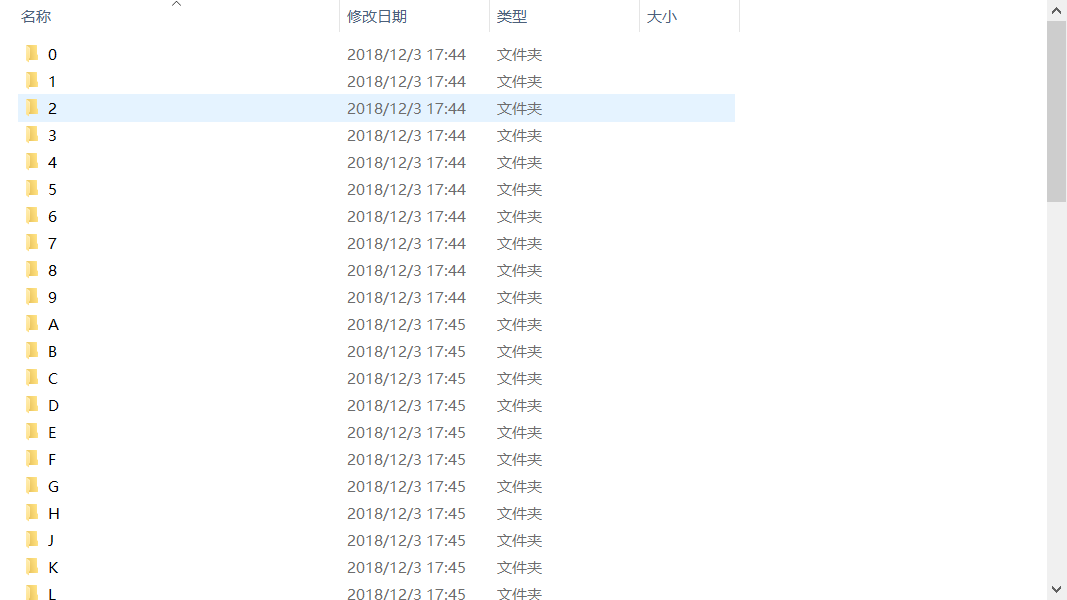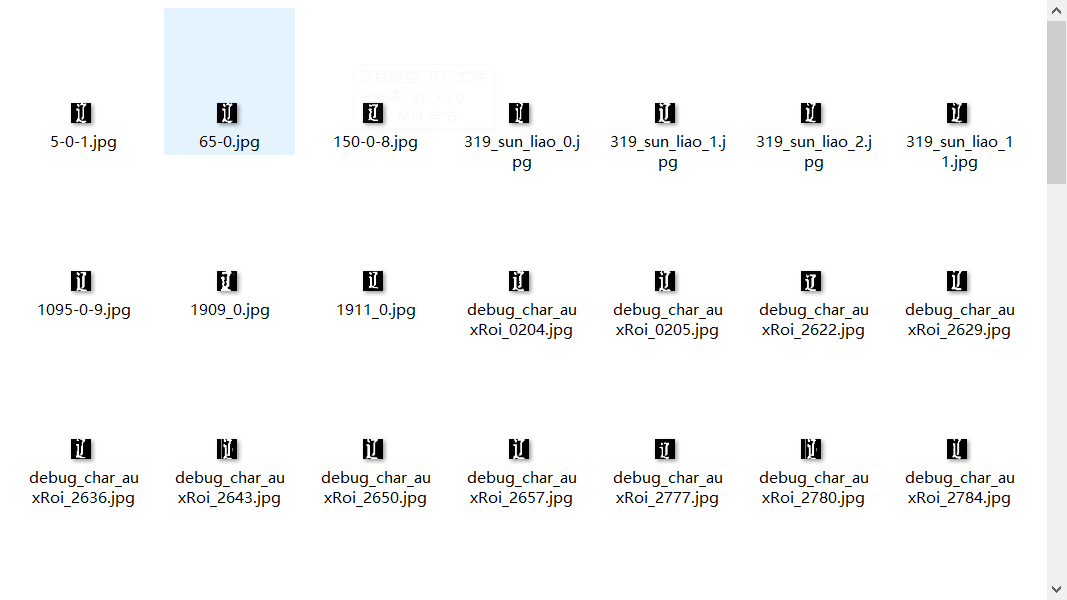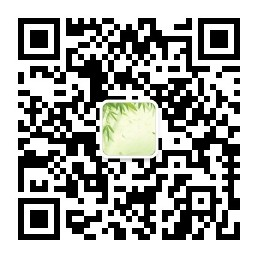# CNN实现车牌识别

16 篇文章 1 订阅

1 数据集准备
1.1 特征提取

1.2 图像处理Fig.1 file condition
2 建模外代码处理
2.1 训练前代码

import sys
import os
import numpy as np
import cv2
import tensorflow as tf
from sklearn.model_selection import train_test_split

numbers = ['0', '1', '2', '3', '4', '5', '6', '7', '8', '9']
alphbets = ['A', 'B', 'C', 'D', 'E', 'F', 'G', 'H', 'I', 'J', 'K', 'L', 'M', 'N', 'O', 'P', 'Q', 'R', 'S', 'T',
'U', 'V', 'W', 'X', 'Y', 'Z']
chinese = ['zh_cuan', 'zh_e', 'zh_gan', 'zh_gan1', 'zh_gui', 'zh_gui1', 'zh_hei', 'zh_hu', 'zh_ji', 'zh_jin',
'zh_jing', 'zh_jl', 'zh_liao', 'zh_lu', 'zh_meng', 'zh_min', 'zh_ning', 'zh_qing', 'zh_qiong',
'zh_shan', 'zh_su', 'zh_sx', 'zh_wan', 'zh_xiang', 'zh_xin', 'zh_yu', 'zh_yu1', 'zh_yue', 'zh_yun',
'zh_zang', 'zh_zhe']

dataset = numbers + alphbets + chinese
dataset_len = len(dataset)
img_size = 20
y_size = len(dataset)
batch_size = 10
x_place = tf.placeholder(dtype=tf.float32, shape=[None, img_size, img_size], name='x_place')
y_place = tf.placeholder(dtype=tf.float32, shape=[None, y_size], name='y_place')
keep_place = tf.placeholder(dtype=tf.float32, name='keep_place')


2.2 训练部分代码

def train(data_dir,save_model_path):
X, y = init_data(data_dir)
print('success load' + str(len(y)) + 'datas')
train_x, test_x, train_y, test_y = train_test_split(X, y, test_size=0.2, random_state=0)

out_put = cnn_construct()
predicts = tf.nn.softmax(out_put)
predicts = tf.argmax(predicts, axis=1)
actual_y = tf.argmax(y_place, axis=1)
accuracy = tf.reduce_mean(tf.cast(tf.equal(predicts, actual_y), dtype=tf.float32))
cost = tf.reduce_mean(tf.nn.softmax_cross_entropy_with_logits(logits=out_put, labels=y_place))
train_step = opt.minimize(cost)

with tf.Session() as sess:
init = tf.global_variables_initializer()
sess.run(init)
step = 0
saver = tf.train.Saver()
while True:
train_index = np.random.choice(len(train_x), batch_size, replace=False)
train_randx = train_x[train_index]
train_randy = train_y[train_index]
_, loss = sess.run([train_step, cost],
feed_dict={x_place:train_randx,y_place:train_randy,keep_place:0.75})
step += 1

if step % 10 == 0:
test_index = np.random.choice(len(test_x), batch_size, replace=False)
test_randx = test_x[test_index]
test_randy = test_y[test_index]
acc = sess.run(accuracy,feed_dict={x_place : test_randx, y_place : test_randy,
keep_place : 1.0})
print(step, loss)
if step % 50 == 0:
print('accuracy:' + str(acc))
if step % 500 == 0:
saver.save(sess, save_model_path, global_step=step)
if acc > 0.99 and step > 500:
saver.save(sess, save_model_path, global_step=step)
break


3 模型参数

def cnn_construct():
x_input = tf.reshape(x_place, shape=[-1, 20, 20, 1])

cw1 = tf.Variable(tf.random_normal(shape=[3, 3, 1, 32], stddev=0.01), dtype=tf.float32)
cb1 = tf.Variable(tf.random_normal(shape=), dtype=tf.float32)
conv1 = tf.nn.max_pool(conv1, ksize=[1, 2, 2, 1], strides=[1, 2, 2, 1], padding='SAME')
conv1 = tf.nn.dropout(conv1, keep_place)

cw2 = tf.Variable(tf.random_normal(shape=[3, 3, 32, 64], stddev=0.01), dtype=tf.float32)
cb2 = tf.Variable(tf.random_normal(shape=), dtype=tf.float32)
conv2 = tf.nn.max_pool(conv2, ksize=[1, 2, 2, 1], strides=[1, 2, 2, 1], padding='SAME')
conv2 = tf.nn.dropout(conv2, keep_place)

cw3 = tf.Variable(tf.random_normal(shape=[3, 3, 64, 128], stddev=0.01), dtype=tf.float32)
cb3 = tf.Variable(tf.random_normal(shape=), dtype=tf.float32)
conv3 = tf.nn.max_pool(conv3, ksize=[1, 2, 2, 1], strides=[1, 2, 2, 1], padding='SAME')
conv3 = tf.nn.dropout(conv3, keep_place)

conv_out = tf.reshape(conv3, shape=[-1, 3 * 3 * 128])

fw1 = tf.Variable(tf.random_normal(shape=[3 * 3 * 128, 1024], stddev=0.01), dtype=tf.float32)
fb1 = tf.Variable(tf.random_normal(shape=), dtype=tf.float32)
fully1 = tf.nn.dropout(fully1, keep_place)

fw2 = tf.Variable(tf.random_normal(shape=[1024, 1024], stddev=0.01), dtype=tf.float32)
fb2 = tf.Variable(tf.random_normal(shape=), dtype=tf.float32)
fully2 = tf.nn.dropout(fully2, keep_place)

fw3 = tf.Variable(tf.random_normal(shape=[1024, dataset_len], stddev=0.01), dtype=tf.float32)
fb3 = tf.Variable(tf.random_normal(shape=[dataset_len]), dtype=tf.float32)
fully3 = tf.add(tf.matmul(fully2, fw3), fb3, name='out_put')

return fully3


4 CNN特征提取
4.1 CNN相对于全连接优势

1、参数数量太多考虑一个输入10001000像素的图片(一百万像素)，输入层有10001000=100万节点。假设第一个隐藏层有100个节点，那么仅这一层就有(1000*1000+1)*100=1亿参数，参数过于庞大！因此可见，图像只扩大一点，参数数量就会多很多，因此它的扩展性很差。
2、没有利用像素之间的位置信息 对于图像识别任务来说，每个像素和其周围像素的联系是比较紧密的，和离得很远的像素的联系可能就很小了。如果一个神经元和上一层所有神经元相连，那么就相当于对于一个像素来说，把图像的所有像素都等同看待，这不符合前面的假设。当我们完成每个连接权重的学习之后，最终可能会发现，有大量的权重，它们的值都是很小的(也就是这些连接其实无关紧要)。努力学习大量并不重要的权重，这样的学习必将是非常低效的。
3、网络层数限制 我们知道网络层数越多其表达能力越强，但是通过梯度下降方法训练深度全连接神经网络很困难，因为全连接神经网络的梯度很难传递超过3层。因此，我们不可能得到一个很深的全连接神经网络，也就限制了它的能力。

1.利用局部连接，这个是最容易想到的，每个神经元不再和上一层的所有神经元相连，而只和一小部分神经元相连。这样就减少了很多参数。
2、权值共享，一组连接可以共享同一个权重，而不是每个连接有一个不同的权重，这样又减少了很多参数。
3,、下采样，可以使用Pooling来减少每层的样本数，进一步减少参数数量，同时还可以提升模型的鲁棒性。

CNN一个非常重要的特点就是头重脚轻（越往输入权值越小，越往输出权值越多），呈现出一个倒三角的形态，这就很好地避免了BP神经网络中反向传播的时候梯度损失得太快。

4.2 三维的层结构

4.2 卷积神经网络的训练

1. 特征不变性，也就是我们在图像处理中经常提到的特征的尺度不变性，池化操作就是图像的resize，平时一张狗的图像被缩小了一倍我们还能认出这是一张狗的照片，这说明这张图像中仍保留着狗最重要的特征，我们一看就能判断图像中画的是一只狗，图像压缩时去掉的信息只是一些无关紧要的信息，而留下的信息则是具有尺度不变性的特征，是最能表达图像的特征。
2. 特征降维，我们知道一幅图像含有的信息是很大的，特征也很多，但是有些信息对于我们做图像任务时没有太多用途或者有重复，我们可以把这类冗余信息去除，把最重要的特征抽取出来，这也是池化操作的一大作用。
3. 在一定程度上防止过拟合，更方便优化

5 总体思路12-09132706-02
04-011万+
03-071万+
08-26419
03-314246
08-20349
06-17489
10-278906
12-051831
04-19
12-18
03-22
10-172873点击重新获取扫码支付余额充值